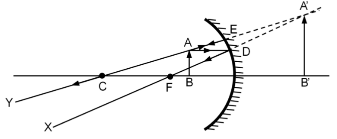# It is desired to obtain an erect image of an object, using concave mirror

It is desired to obtain an erect image of an object, using concave mirror of focal length of 12cm.

a) What should be the range of distance of an object placed in front of the mirror?
b) Will the image be smaller or larger than the object. Draw ray diagram to show the formation of image in this case.
c) Where will the image of this object be, if it is placed 24 cm in front of the mirror? Draw ray diagram for this situation.
Show the positions of pole, principal focus and the centre of curvature in the above ray diagrams.

a) In concave mirror erect is formed only when the object is placed between pole and focus. So object distance should be less than 12 cm
b) The image would be larger than the objectc) If the object distance is 24 cm which is the approx position of centre of curvature, then the image will be formed at the same position